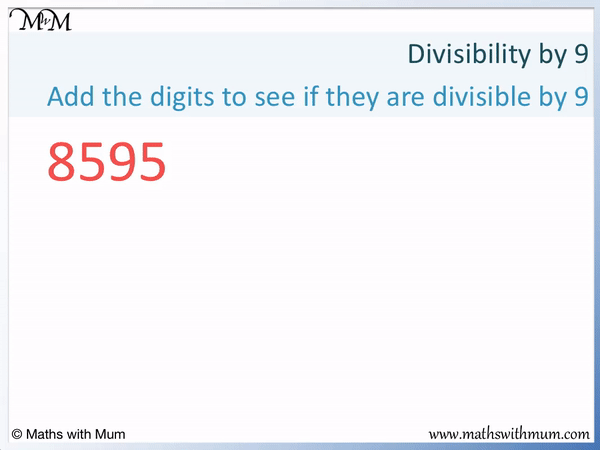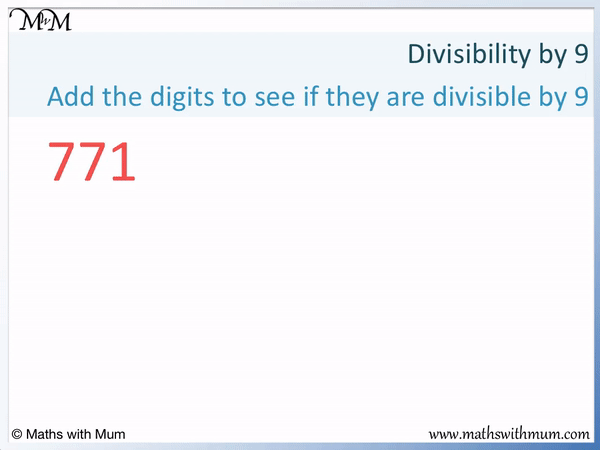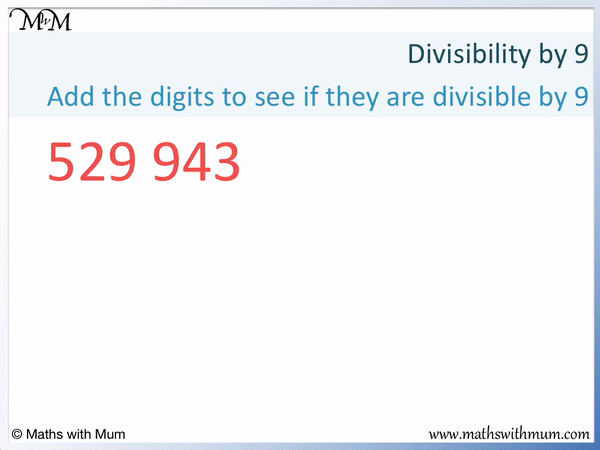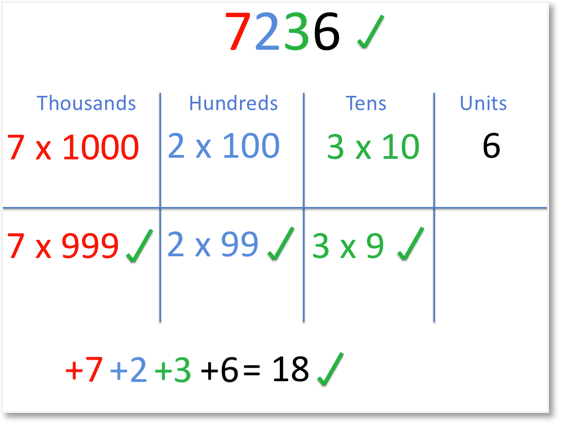# How to Tell if a Number is Divisible by 9

How to Tell if a Number is Divisible by 9• If a number is divisible by 9, it means that it can be divided exaclty by 9 with no remainder.
• A number is divisible by 9 if the sum of its digits is also divisible by 9.
• Add the digits of the number and decide if this total can be divided exactly by 9.
• 8 + 5 + 9 + 5 = 27.
• 27 = 9 × 3 and so, 27 is divisible by 9.
• 27 is divisible by 9 therefore 8595 is also divisible by 9.
• This trick works for all numbers, no matter how large.
• If the sum of the digits is not divisible by 9, then the number isn’t either.
• We can use the same trick to decide if the sum of the digits is divisble by 9 if we are not sure.
• In this example, 2 + 7 = 9, which tells us that 27 is divisible by 9.
A number is divisible by 9 if the sum of its digits is also divisible by 9.• A number is divisible by 9 if the sum of its digits is divisible by 9.
• We add the digits of 409.
• 4 + 0 + 9 = 13.
• 13 is not divisible by 9. It is not in the 9 times table.
• 13 is not divisible by 9 and so, 409 is not divisible by 9 either.Supporting Lessons

#### Divisibility by 9# Divisibility by 9

## How to Tell if a Number is Divisible by 9

To decide if a number is divisible by 9, follow these steps:

1. Add the digits of the number.
2. If the sum of these digits is divisible by 9, the original number is divisible by 9.
3. If the sum of these digits is not divisible by 9, the original number is not divisible by 9.
4. Use steps 1 to 3 again to decide if the sum of the digits of the number are divisible by 9.If a number is divisible by 9 it means that the number can be divided by 9 exactly leaving no remainder.

The rule for divisibility by 9 is that the number is divisible by 9 only if the sum of its digits is also divisible by 9.

In this example, we will use the rule for divisibility by 9 to test if 8595 is divisible by 9.

The first step is to add the digits of the number.

8 + 5 + 9 + 5 = 27.The next step is to decide if the sum of the digits of the number are divisible by 9.

27 is divisible by 9 because it is 9 × 3.If the sum of the digits is divisible by 9, the number itself is divisble by 9.

27 is divisible by 9 and so, 8595 is also divisible by 9.

If we are unsure whether the sum of the digits is divisible by 9, add the digits of this number and decide if the new total is also divisible by 9.

For example, here 27 is divisible by 9 because 2 + 7 = 9.

Here is an example of using the rule for divisibility by 9. We will check if 771 is divisible by 9.

The rule to check divsibility by 9 is to add the digits and decide if this total is divisible by 9.

7 + 7 + 1 = 15

15 is not a multiple of 9. The first two multiples of 9 are 9 and 18.15 is not divisible by 9 and so, 771 is not divisible by 9. A number is only divisible by 9 if its digits add to a number that is divisible by 9.It does not matter how large the number is. The rule for divisibility by 9 always works without exception.

For example, here is 7,719,984.

7 + 7 + 1 + 9 + 9 + 8 + 4 = 45

45 is divisible by 9 and so, 7,719,984 is also divisible by 9.Here is a large example of a number not divisible by 9. Here is 529,943.5 + 2 + 9 + 9 + 4 + 3 = 32

32 is not divisible by 9 and so, 529,943 is not divisible by 9

## Why Does the Rule for Divisibility by 9 Work?

The rule for divisibility by 9 works because numbers are written in base 10. Each digit of a number represents a multiple of 9 plus the value of that digit. The multiple of 9 is divisible by 9 so only the value of the digits needs to be checked. A number is divisible by 9 if the sum of its digits is divisible by 9.

For example, 7236 can be written as 7 × 1000 plus 2 × 100 plus 3 × 10 plus 6.7 × 1000 is the same as 7 × 999 + 7, 2 × 100 is the same as 2 × 99 + 2 and 3 × 10 is the same as 3 × 9 + 3.The multiples of 999, 99 and 9 are divisible by 9 and so, we just need to check the remaining digits.If 7 + 2 + 3 + 6 is divisible by 9, then the whole number is.

7 + 2 + 3 + 6 = 18, which is in the 9 times table. Therefore 7236 is divisible by 9.## List of Numbers Divisible by 9

There are 11 numbers less than 100 that are divisible by 9:

9, 18, 27, 36, 45, 63, 72, 81, 90 and 99.

The numbers divisible by 9 between 1 and 100 are easy to remember because their digits add up to 9.Now try our lesson on Finding the Mean where we learn how to calculate the mean of a set of numbers.error: Content is protected !!